Showing first {{hits.length}} results of {{hits_total}} for {{searchQueryText}}{{hits.length}} results for {{searchQueryText}}

Tikz is probably the most complex and powerful tool to create graphic elements in LaTeX. In this article some of the basics will be explained: lines, dots, curves, circles, rectangles, etc by means of simple examples. Contents 1 Introduction 2 Basic elements: points, lines and paths 3 Basic geometric shapes: Circles, ellipses and polygons 4 Diagrams 5 Reference Guide 6 Further reading  Introduction We can create graphic elements easily by defining some of their key properties, let's see: \begin{tikzpicture} \draw[gray, thick] (-1,2) -- (2,-4); \draw[gray, thick] (-1,-1) -- (2,2); \filldraw[black] (0,0) circle (2pt) node[anchor=west] {Intersection point};   \end{tikzpicture}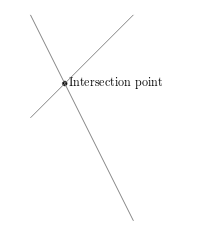First, you declare a tikzpicture environment, before this you must include the line \usepackage{tikz} in the preamble of your document. In this example two lines and one point are drawn. To add a line the command \draw[gray, thick] defines a graphic element whose colour is gray and with a thick stroke. The line is actually defined by it's two endpoints, (-1,2) and (2,-4), joined by --. The point is actually a circle drawn by \filldraw[black], this command will not only draw the circle but fill it with black colour. In this command the centre point (0,0) and the radius (2pt) are declared. Next to the point is a node, which is actually a box containing the "intersection point" text, is anchored at the west of the point. It's important to notice the semicolon ; at the end of each draw command. Note: The tikzfigure environment can be enclosed inside a figure or similar environment. See the Inserting Images article for more information about this subject.   Open an example of the tikz package in Overleaf  Basic elements: points, lines and paths In this section is explained how to create basic graphic elements. These elements can be combined to create more elaborated figures. \begin{tikzpicture}   \draw (-2,0) -- (2,0); \filldraw [gray] (0,0) circle (2pt); \draw (-2,-2) .. controls (0,0) .. (2,-2); \draw (-2,2) .. controls (-1,0) and (1,0) .. (2,2);   \end{tikzpicture}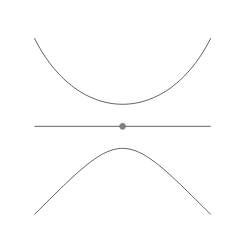There are three basic commands in this example: \draw (-2,0) -- (2,0); This defines a line whose endpoint are (-2,0) and (2,0). \filldraw [gray] (0,0) circle (2pt); The point is created as a very small gray circle centred at (0,0) and whose radius is (2pt). The \filldraw command is used in to draw elements and fill them with some specific colour. See the next section for more examples. \draw (-2,2) .. controls (-1,0) and (1,0) .. (2,2); Draws a Bézier curve, is a bit tricky at first. There are 4 points defining it: (-2,2) and (2,2) are its endpoints, (-1,0) and (1,0) are control points (can be equal) that determine 'how curved' it is. You can think of these two points as "attractor points".   Open an example of the tikz package in Overleaf  Basic geometric shapes: Circles, ellipses and polygons Geometric figures can be made up from simpler elements or created by an special command. Let's start with circles, ellipses and arcs. \begin{tikzpicture} \filldraw[color=red!60, fill=red!5, very thick](-1,0) circle (1.5); \fill[blue!50] (2.5,0) ellipse (1.5 and 0.5); \draw[ultra thick, ->] (6.5,0) arc (0:220:1); \end{tikzpicture}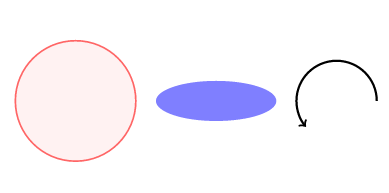\filldraw[color=red!60, fill=red!5, very thick](-1,0) circle (1.5); We are already familiar with this command, it was used to draw a point in the previous section, but here there are some additional parameters inside brackets. These are explained below. color=red!60 The colour of the ring around the circle is set to 60% red (lighter than only red). See the reference guide for a list of the default colours available in LaTeX; also, see Using colours in LaTeX to learn how to create your own colours. fill=red!5 The circle is filled in with an even more lighter red. very thick This parameter defines the thickness of the stroke, can also be used in the \fill command. See the reference guide for a complete list of values. \fill[blue!50] (2.5,0) ellipse (1.5 and 0.5); To create an ellipse you provide a centre point (2.5,0), and two radii: horizontal and vertical (1.5 and 0.5). Also notice the command fill instead of draw or filldraw, this is because in this case there's no need to control outer and inner colours. \draw[ultra thick, ->] (6.5,0) arc (0:220:1); This command will draw an arc, the extra parameter -> indicates that the arc will have an arrow at the end. To draw the arc you must provide the centre point and the other three numbers: the starting and ending angles, and the radius; in the format (0:220:1). But not only curved geometric elements can be created. Straight-edged ones use a similar syntax: \begin{tikzpicture}   \draw[blue, very thick] (0,0) rectangle (3,2); \draw[orange, ultra thick] (4,0) -- (6,0) -- (5.7,2) -- cycle;   \end{tikzpicture}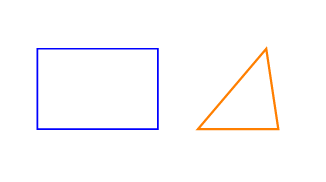\draw[blue, very thick] (0,0) rectangle (3,2); Rectangles are created by the special command rectangle. You have to provide two points, the first one is where the "pencil" begins the draw and the second one is the diagonally opposite corner point. \draw[orange, ultra thick] (4,0) -- (6,0) -- (5.7,2) -- cycle; To draw a polygon we draw a closed path of straight lines: a line from (4,0) to (6,0) and a line from (6,0) to (5.7,2). Finally, the last point declared is not such thing, but the word cycle, meaning the next line should join the last and the first points.   Open an example of the tikz package in Overleaf  Diagrams The nodes are probably the most versatile elements in Tikz. We've already used one node in the introduction to add some text to the figure. In the next example nodes will be used to create a diagram. \begin{tikzpicture}[ roundnode/.style={circle, draw=green!60, fill=green!5, very thick, minimum size=7mm}, squarednode/.style={rectangle, draw=red!60, fill=red!5, very thick, minimum size=5mm}, ] %Nodes \node[squarednode] (maintopic) {2}; \node[roundnode] (uppercircle) [above=of maintopic] {1}; \node[squarednode] (rightsquare) [right=of maintopic] {3}; \node[roundnode] (lowercircle) [below=of maintopic] {4};   %Lines \draw[->] (uppercircle.south) -- (maintopic.north); \draw[->] (maintopic.east) -- (rightsquare.west); \draw[->] (rightsquare.south) .. controls +(down:7mm) and +(right:7mm) .. (lowercircle.east); \end{tikzpicture}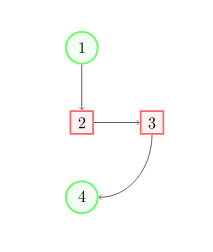There are essentially three commands in this figure: A node definition, a node declaration and lines that join two nodes. roundnode/.style={circle, draw=green!60, fill=green!5, very thick, minimum size=7mm} Passed as a parameter to the tikzpicture environment defines a node that will be referred as roundnode, this node will be a circle whose outer ring will be gren!60 and will be coloured with green!5, the stroke will be very thick and its minimum size is 7mm. The line below this defines a second rectangle-shaped node called squarednode with similar parameters. \node[squarednode] (maintopic) {2}; This will create a squarednode, as defined in the previous command. This node will have an id, maintopic and will contain the number 2, if you leave an empty space inside the braces no text will be displayed. [above=of maintopic] Notice that all but the first node have an additional parameter, this parameter determines its position relative to other node. For instance [above=of maintopic] means that this node should appear above the node named maintopic. For this positioning system to work you have to add \usetikzlibrary{positioning} to your preamble. Without the positioning library, you can use the syntax above of=maintopic instead, but the positioning syntax is more flexible and powerful: you can extend it to write above=3cm of maintopic i.e. control the actual distance from maintopic. \draw[->] (uppercircle.south) -- (maintopic.north); An arrow-like straight line will be drawn. The syntax has been already explained at the basic elements section. The only thing special is the manner we write the endpoints of the line, by referencing a node (this is why we named them) and a position relative to the node.   Open an example of the tikz package in Overleaf  Reference Guide Possible color and thickness parameters in the tikz package: parameter values picture color white, black, red, green, blue, cyan, magenta, yellow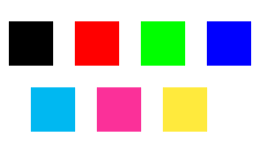thickness ultra thin, very thin, thin, thick, very thick, ultra thick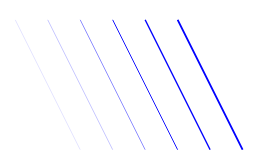More colours may be available in your LaTeX distribution. See Using colours in LaTeX  Further reading For more information see: Using colours in LaTeX Pgfplots package Inserting Images Lists of tables and figures Positioning images and tables Drawing diagrams directly in LaTeX The TikZ and PGF Packages: Manual for version 3.0.0 TikZ and PGF examples at TeXample.net